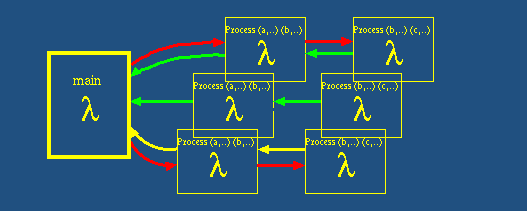Project Goals

# SPICA Project

This project aims for a Skeleton-based Parallel Implementation of selected Computer Algebra Algorithms in Eden.

## Project Goal

The goal of this project is an abstract, mathematically profound specification and a parallel implementation of major algorithms of computer algebra. Such algorithms describe algebraic, exact, symbolic computations. Although an implementation in a functional programming language promises deep connections to the mathematical background, most implementations are imperative for the efficiency. However such approach blocks a flexible development and hence -- an easy parallelization for multicores or grids. This projects selects few algorithms of computer algebra:

• basic operations, such as
• fast polynomial multiplication
• fast matrix multiplication
• variants of the euclidean algorithm
• methods of multiple residue arithmetic
• probabilistic prime testing
• Buchberger's algorithm

for a parallel implementation in Eden. The latter is a parallel extension of parallel functional language Haskell. A special focus is set on abstracting the fitting computation schemes to algorithmic skeletons. Such parallel generalized computation schemes are expressed as higher order functions and can be utilized for parallelizing whole classes of algorithms. Usage of highly-optimized skeletons present a prospect of precision, flexibility, efficiency and versatility. As a whole, this project leads to the foundations of a flexible open parallel computer algebra system.

## Project Timeline

The project funding by DFG began in April 2009. As of now, skeletons and their instantiations are written and available for the following algorithms.

• FFT, for fast polynomial multiplication
• Strassen's algorithm for matrix multiplication
• LU factorisation of matrices
• integral and fractional multiple-residue arithmetic
• includes variants of euclidean algorithm for integers
• Rabin-Miller primality test
• APRCL primality test
• Buchberger's algorithm
• sequential variant available
• parallel version and skeleton in work

More information on skeletons, including the source code, is available on the designated page.

## Trivia

Spica is the 15th brightest star, 260 light years away from the Earth. Hipparchus is believed to have observed it to discover precession of the equinoxes. To locate Spica at nighttime follow the arc of the handle of the Big Dipper to Arcturus and follow it forth for the same distance once more.

The star name comes from Latin "ear of wheat (in the hand of the goddess)".Oleg Lobachev. E-Mail
Fb. 12 - Mathematik und Informatik, Hans-Meerwein-Straße, D-35032 Marburg